Test: Problems Based On Figures- 2

# Test: Problems Based On Figures- 2 - Class 9

Test Description

## 15 Questions MCQ Test Cyber Olympiad for Class 9 - Test: Problems Based On Figures- 2

Test: Problems Based On Figures- 2 for Class 9 2023 is part of Cyber Olympiad for Class 9 preparation. The Test: Problems Based On Figures- 2 questions and answers have been prepared according to the Class 9 exam syllabus.The Test: Problems Based On Figures- 2 MCQs are made for Class 9 2023 Exam. Find important definitions, questions, notes, meanings, examples, exercises, MCQs and online tests for Test: Problems Based On Figures- 2 below.
Solutions of Test: Problems Based On Figures- 2 questions in English are available as part of our Cyber Olympiad for Class 9 for Class 9 & Test: Problems Based On Figures- 2 solutions in Hindi for Cyber Olympiad for Class 9 course. Download more important topics, notes, lectures and mock test series for Class 9 Exam by signing up for free. Attempt Test: Problems Based On Figures- 2 | 15 questions in 30 minutes | Mock test for Class 9 preparation | Free important questions MCQ to study Cyber Olympiad for Class 9 for Class 9 Exam | Download free PDF with solutions
 1 Crore+ students have signed up on EduRev. Have you?
Test: Problems Based On Figures- 2 - Question 1

### Choose the code letters that you think describes the shape in the box on the right hand side and circle the letter on the answer sheet, or mark the appropriate box on the multiple choice answer sheet. Which figure completes the series?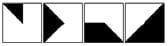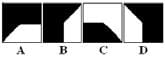Test: Problems Based On Figures- 2 - Question 2

### Choose the code letters that you think describes the shape in the box on the right hand side and circle the letter on the answer sheet, or mark the appropriate box on the multiple choice answer sheet. Which figure completes the series?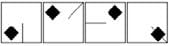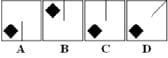Test: Problems Based On Figures- 2 - Question 3

### Choose the code letters that you think describes the shape in the box on the right hand side and circle the letter on the answer sheet, or mark the appropriate box on the multiple choice answer sheet. Which figure completes the series?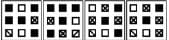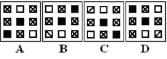Test: Problems Based On Figures- 2 - Question 4

Choose the code letters that you think describes the shape in the box on the right hand side and circle the letter on the answer sheet, or mark the appropriate box on the multiple choice answer sheet.

Which figure completes the series?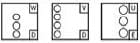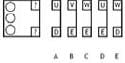Test: Problems Based On Figures- 2 - Question 5

Choose the code letters that you think describes the shape in the box on the right hand side and circle the letter on the answer sheet, or mark the appropriate box on the multiple choice answer sheet.

Which figure completes the series?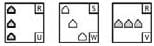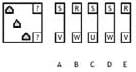Test: Problems Based On Figures- 2 - Question 6

Choose the code letters that you think describes the shape in the box on the right hand side and circle the letter on the answer sheet, or mark the appropriate box on the multiple choice answer sheet.

Which figure completes the series?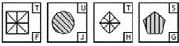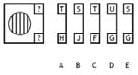Test: Problems Based On Figures- 2 - Question 7

Choose the code letters that you think describes the shape in the box on the right hand side and circle the letter on the answer sheet, or mark the appropriate box on the multiple choice answer sheet.

Which figure completes the series?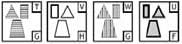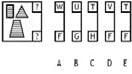Test: Problems Based On Figures- 2 - Question 8

Choose the code letters that you think describes the shape in the box on the right hand side and circle the letter on the answer sheet, or mark the appropriate box on the multiple choice answer sheet.

Which figure completes the series?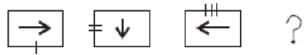Test: Problems Based On Figures- 2 - Question 9

Choose the code letters that you think describes the shape in the box on the right hand side and circle the letter on the answer sheet, or mark the appropriate box on the multiple choice answer sheet.

Which figure completes the series?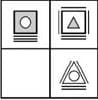Test: Problems Based On Figures- 2 - Question 10

Choose the code letters that you think describes the shape in the box on the right hand side and circle the letter on the answer sheet, or mark the appropriate box on the multiple choice answer sheet.

Which figure completes the series?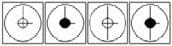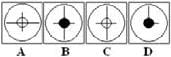Test: Problems Based On Figures- 2 - Question 11

Choose the code letters that you think describes the shape in the box on the right hand side and circle the letter on the answer sheet, or mark the appropriate box on the multiple choice answer sheet.

Select a suitable figure from the four alternatives that would complete the figure matrix.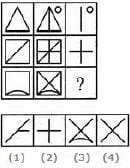Detailed Solution for Test: Problems Based On Figures- 2 - Question 11

The third figure in each row comprises of parts which are not common to the first two figures.

Test: Problems Based On Figures- 2 - Question 12

Choose the code letters that you think describes the shape in the box on the right hand side and circle the letter on the answer sheet, or mark the appropriate box on the multiple choice answer sheet.

Select a suitable figure from the four alternatives that would complete the figure matrix.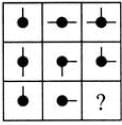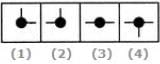Detailed Solution for Test: Problems Based On Figures- 2 - Question 12

In each row, the third figure comprises of a black circle and only those line segments which are not common to the first and the second figures.

Test: Problems Based On Figures- 2 - Question 13

Choose the code letters that you think describes the shape in the box on the right hand side and circle the letter on the answer sheet, or mark the appropriate box on the multiple choice answer sheet.

Select a suitable figure from the four alternatives that would complete the figure matrix.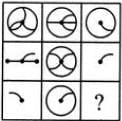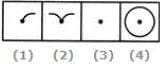Detailed Solution for Test: Problems Based On Figures- 2 - Question 13

The third figure in each row comprises of the parts common to the first two figures.

Test: Problems Based On Figures- 2 - Question 14

Choose the code letters that you think describes the shape in the box on the right hand side and circle the letter on the answer sheet, or mark the appropriate box on the multiple choice answer sheet.

Select a suitable figure from the four alternatives that would complete the figure matrix.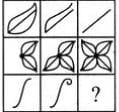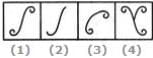Detailed Solution for Test: Problems Based On Figures- 2 - Question 14

The number of components in each row either increases or decreases from left to right. In the third row, it increases.

Test: Problems Based On Figures- 2 - Question 15

Choose the code letters that you think describes the shape in the box on the right hand side and circle the letter on the answer sheet, or mark the appropriate box on the multiple choice answer sheet.

Select a suitable figure from the four alternatives that would complete the figure matrix.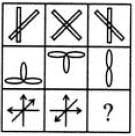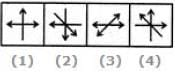Detailed Solution for Test: Problems Based On Figures- 2 - Question 15

The third figure in each row comprises of parts which are not common to the first two figures.

## Cyber Olympiad for Class 9

13 docs|50 tests
Information about Test: Problems Based On Figures- 2 Page
In this test you can find the Exam questions for Test: Problems Based On Figures- 2 solved & explained in the simplest way possible. Besides giving Questions and answers for Test: Problems Based On Figures- 2, EduRev gives you an ample number of Online tests for practice

## Cyber Olympiad for Class 9

13 docs|50 tests(Scan QR code)